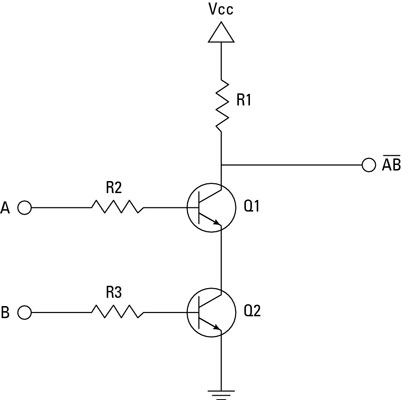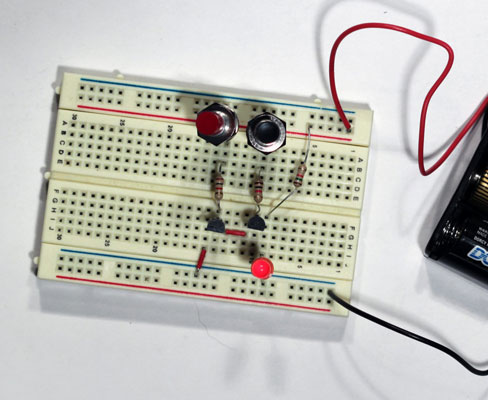## Use Transistors to Build a NAND Gate

A two-input NAND gate produces a LOW output if both of its inputs are HIGH, and a HIGH output otherwise. It's easy enough to create a NAND gate by using just two transistors. Consider the circuit diagram below.This is easily implemented on a solderless breadboard. We can use open pushbuttons for the two inputs, $A$ and $B$. We can have a nice visual representation of the output by placing a light-emitting diode (LED) at the point marked $\overline{AB}$.

If both of the inputs are HIGH, both of the transistors will conduct through their collector-emitter paths (i.e., the north-to-south path in the diagram), which creates a short circuit to ground. This causes the current to bypass the output altogether, which in turn causes the output to go LOW.

If either transistor turns off, however, the supply current can't flow through the transistors to ground, so it flows through the output circuit instead. Thus, the output is HIGH if either one of the inputs is LOW. If both inputs are HIGH, the output is LOW.

Consequently, the LED will be on until you press both of the pushbuttons. This action causes both inputs to go HIGH, which causes the output to go LOW and the LED to go dark.Original illustrations and text by Doug Lowe, adapted by P. Oser